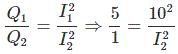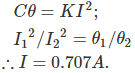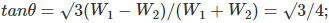# MCQs on Electrical And Electronics Measurements

##### Page 12 of 19. Go to page 1 2 3 4 5 6 7 8 9 10 11 12 13 14 15 16 17 18 19
01․ For measuring an AC voltage by an AC potentiometer it is desirable that the supply for the potentiometer is taken from
A battery.
The same source as the unknown voltage.
A source other than the source of unknown voltage.
Any of above.

For satisfactory operation of an AC potentiometer, the potentiometer circuit should be supplied from the same source as the unknown voltage or current being measured.

02․ The transfer instrument which is used for standardization of a polar type AC potentiometer is
an electrostatic instrument.
a moving coil instrument.
a thermal instrument.
a dynamometer instrument.

A thermal instrument (thermocouple type) is used as a transfer instrument for standardization of a polar type AC potentiometer. Such an instrument can be calibrated on DC and then brought to the same setting on AC.

03․ Standardization of potentiometer is done in order that, they become
accurate.
precise.
accurate and precise.

The standardization is done with help of a standard DC source i.e. a standard cell or Zener source and a transfer instrument. The AC potentiometer is made direct reading type i.e. the reading is read off directly from the dial setting. So standardization is done in order to make the instrument more accurate and direct reading.

04․ A DC potentiometer is the best means available for the measurement of DC voltage because
It is based on null balance technique.
It is possible to standardize before a measurement is undertaken.
The precision in measurment is independent of the type of the defector used.
None of above.

A DC potentiometer is the best means available for the measurement of DC voltage because it is based on null balance technique and it is possible to mesure DC voltage ranging in value from mV to hundreds of volts.

05․ A thermo couple ammeter gives full scale deflection of 10 A, when it reads one fifth of the scale, the current will be
2 A.
4.47 A.
4 A.
5.78 A.⇒ I2 = 4.47 A.

06․ A meter has a full-scale deflection of 90o at a current of 1 A. The response of the meter is square law. Assuming spring control, the current for a deflection at 45o will be
0.25 A.
0.50 A.
0.70 A.
0.67 A.

We know that TD = Cθ ............(1) Also TD = KI2..............(2) From equation (1) and (2)07․ Ratio of readings of two wattmeter connected to measure power in a balanced 3-phase load is 5:3 and the load is inductive. The power factor at load is
0.6 lag.
0.917 lag.

We know,Cosθ = 0.917; as the circuit is inductive, the pf will be lagging.

08․ Magnetic deflection is inversely proportional to
Voltage.
(Voltage)-0.5.
(Voltage)1.5.
(Voltage)2.

Magnetic deflection is to observe the effect of a magnetic field on an electron beam in a CRO. It is inversely proportional to the square root of the potential difference between anode and cathode.

09․ How many time-base circuits does a dual face CRO can have?
1.
2.
3.
4.

Dual face oscilloscope uses a single electron gun and produces multiple traces by switching the y-deflection plates from one input signal to another.

10․ A 1 mA meter is to be converted to 1 A ammeter. The meter resistance is 100 Ω. The value of shunt resistance
0.001 Ω.
0.1001 Ω.
100 Ω.
1000 Ω.

Shunt resistance = 0.001 × 100/(1 – 0.001) = 0.1001 Ω.

<<<1011121314>>>